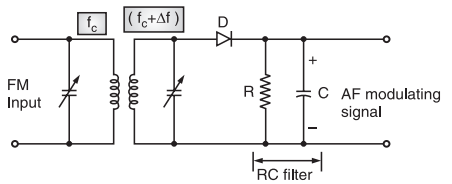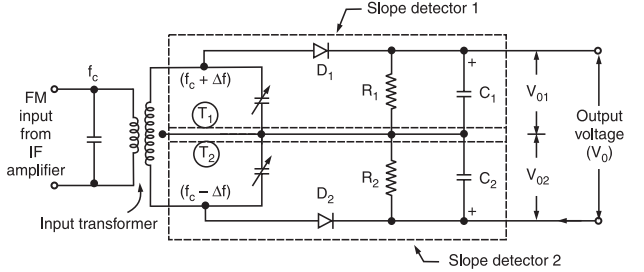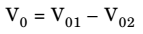# FM Slope Detector

### Simple FM Slope Detector

The circuit diagram of a simple slope detector is as shown in figure 1.Fig.1 : Simple Slope Detector

The output voltage of the tank circuit is then applied to a simple diode detector of an RC load with proper time constant.

This detector is identical to the AM diode detector. Even though the slope detector circuit is simple it has the following drawbacks.

#### Drawbacks of Slope Detector

(i) It is inefficient.

(ii) It is linear only over a limited frequency range.

(iii) It is difficult to adjust as the primary and secondary winding of the transformer must be tuned to slightly different frequencies.

The only advantages of the basic slope detector circuit is its simplicity.

To overcome the drawbacks of the simple slope detector, a Balanced slope detector is used.

### Balanced FM Slope Detector (Balanced Frequency Discriminator)

The circuit diagram of the balanced slope detector is shown in Figure. 2.Fig.2 : Balanced Slope Detector

As shown in the circuit diagram, the balanced slope detector consists of two slope detector circuits.

The input transformer has a center tapped secondary. Hence, the input voltages to the two slope detectors are 180° out of phase.

There are three tuned circuits.

Out of them, the primary is tuned to IF i.e., fc .

The upper tuned circuit of the secondary (T1) is tuned above fc  by Δf i.e., its resonant frequency is (fc+ Δf).

The lower tuned circuit of the secondary is tuned below fc by Δf i.e., at (fc – Δf).

R1C1 and R2C2 are the filters used to bypass the RF ripple.

Vo1 and Vo2 are the output voltages of the two slope detectors.

The final output voltage Vo is obtained by taking the subtraction of the individual output voltages, Vo1 and Vo2, i.e.,#### Working Operation of the Circuit

The circuit operation can be explained by dividing the input frequency into three ranges as follows:

(i) fin = fc: When the input frequency is instantaneously equal to fc, the induced voltage in the T1 winding of secondary is exactly equal to that induced in the winding T2.

Thus, the input voltages to both the diodes D1 and D2 will be the same.

Therefore, their dc output voltages Vo1 and Vo2 will also be identical but they have opposite polarities. Hence, the net output voltage Vo = 0.

(ii) fc < fin < (fc + Δf): In this range of input frequency, the induced voltage in the winding T1 is higher than that induced in T2.

Therefore, the input to D1 is higher than D2.

Hence, the positive output Vo1 of D1 is higher than the negative output Vo2 of D2.

Therefore, the output voltage Vo is positive.

As the input frequency increases towards (fc + Δf), the positive output voltage increases as shown in 3.Fig.3: Characteristics of the balanced slope detector

If the output frequency goes outside the range of (fc – Δf) to (fc + Δf), the output voltage will fall due to the reduction in tuned circuit response.